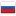Codeforces Round #367 (Editorial)

Revision en7, by NBAH, 2016-08-12 14:10:10

## A

We know that time=distance/speed. For each car we should find timei, than if it is less than answer we should update it.

Time Complexity: O(n).

Solution

## B

Consider c[x] the number of stores in which the price per drink is x. We calculate this array prefix sum. Then the following options:

1) If the current amount of money m is larger than the size of the array with the prefix sums than answer is n.

2) Otherwise, the answer is c[m].

Time Complexity: O(n+q).

Solution

## C

We will solve the problem with the help of dynamic programming. dp[i][j] is the minimum amount of energy that should be spent to make first i strings sorted in lexicographical order and i-th of them will be reversed if j = 1 and not reversed if j = 0. dp[i][j] is updated by dp[i - 1] and dp[i - 1]. It remains to verify that the i-th string is lexicographically greater than (i - 1)-th (if j = 1 then we should check reversed i-th string, similar to (i - 1)-th). Then we update dp[i][j] = min(dp[i][j], dp[i - 1] + c[i] * j), dp[i][j] = min(dp[i][j], dp[i - 1] + j * c[i]). The answer is a minimum of dp[n] and dp[n].

Time Complexity: O(n+sum_length).

Solution

## D

Let's store each number in binary system (each number consists of 32 bits, 0 or 1) in such a data structure as trie.The edges will be the bits 1 and 0, and the vertices will be responsible for whether it is possible to pass the current edge. To reply to a query like "? X" will descend the forest of high-order bits to whether the younger and now we can look XOR in the i-th bit to get one, if we can, then move on, otherwise we go to where we can go.

Time Complexity: O(q*log(10^9)).

Solution

## E

Let's surround the matrix with the frame of elements. In each element of the matrix, and frame we need to store value, the number of the right element and the number of down element. When a request comes we should change only values of the elements along the perimeter of rectangles.

Time Complexity: O(q*(n+m)).

Solution

#### History

RevisionsRev. Lang. By When Δ Comment
ru7NBAH 2016-08-12 14:14:05 49
ru6NBAH 2016-08-12 14:13:31 192
en7NBAH 2016-08-12 14:10:10 241
ru5NBAH 2016-08-12 14:01:30 340
en6NBAH 2016-08-12 14:00:03 345
ru4NBAH 2016-08-12 11:17:18 60
ru3NBAH 2016-08-12 11:15:17 6
en5NBAH 2016-08-12 11:13:36 4
en4NBAH 2016-08-12 11:13:14 54
en3NBAH 2016-08-12 11:07:57 4 Tiny change: ' can look RRF in the i-' -> ' can look XOR in the i-'
ru2NBAH 2016-08-11 23:50:55 8 Мелкая правка: 'оседнего слева. Когда пр' -> 'оседнего снизу. Когда пр'
en2NBAH 2016-08-11 23:50:19 541 (published)
en1NBAH 2016-08-11 23:45:01 2129 Initial revision for English translation (saved to drafts)
ru1NBAH 2016-08-11 22:31:18 2288 Первая редакция (опубликовано)